50 MHz to 4.0 GHz
RF/IF Gain Block
Data Sheet
Rev. A Document Feedback
Information furnished by Analog Devices is believed to be accurate and reliable. However, no
responsibility is assumed by Analog Devices for its use, nor for any infringements of patents or other
rights of third parties that may result from its use. Specifications subject to change without notice. No
license is granted by implication or otherwise under any patent or patent rights of Analog Devices.
One Technology Way, P.O. Box 9106, Norwood, MA 02062-9106, U.S.A.
Technical Support www.analog.com
FEATURES
Fixed gain of 20 dB
Operation from 50 MHz to 4.0 GHz
Highest dynamic range gain block
Input/output internally matched to 50 Ω
Integrated bias control circuit
OIP3 of 42.0 dBm at 2.0 GHz
P1dB of 19.3 dBm at 2.0 GHz
Noise figure of 3.3 dB at 2.0 GHz
Single 5 V power supply
Low quiescent current of 89 mA
Thermally efficient SOT-89 package
ESD rating of ±1.5 kV (Class 1C)
FUNCTIONAL BLOCK DIAGRAM
RFIN GND RFOUT
1 2
BIAS
3
GND
(2)
08190-001
Figure 1.
GENERAL DESCRIPTION
at frequencies up to 4 GHz. The device can be used in a wide
variety of cellular, CATV, military, and instrumentation equipment.
The ADL5602 provides the highest dynamic range available
from an internally matched gain block. This is accomplished
by providing extremely low noise figures and very high OIP3
specifications simultaneously, across the entire 4 GHz
frequency range.
The ADL5602 provides a gain of 20 dB, which is stable over
frequency, temperature, power supply, and from device to
device. The device is internally matched to 50 Ω at the input
and output, making the ADL5602 very easy to implement in a
wide variety of applications. Only input/output ac coupling
capacitors, power supply decoupling capacitors, and an external
inductor are required for operation.
The ADL5602 is fabricated on an InGaP HBT process and has
an ESD rating of ±1.5 kV (Class 1C). The device is available in a
thermally efficient SOT-89 package.
The ADL5602 consumes 89 mA on a single 5 V supply and is
fully specified for operation from −40°C to +8C.
A fully populated RoHS-compliant evaluation board is available.Rev. A | Page 2 of 16
Features .............................................................................................. 1
Functional Block Diagram .............................................................. 1
General Description ......................................................................... 1
Revision History ............................................................................... 2
Specifications ..................................................................................... 3
Typical Scattering Parameters (S Parameters) .......................... 5
Absolute Maximum Ratings ............................................................ 7
ESD Caution .................................................................................. 7
Pin Configuration and Function Descriptions ............................. 8
Typical Performance Characteristics ............................................. 9
Basic Connections .......................................................................... 12
Soldering Information and Recommended
PCB Land Pattern ....................................................................... 12
W-CDMA ACPR Performance .................................................... 13
Evaluation Board ............................................................................ 14
Outline Dimensions ....................................................................... 15
Ordering Guide .......................................................................... 15
REVISION HISTORY
11/13—Rev. 0 to Rev. A
Changes to Figure 2 ........................................................................... 8
Added Figure 15, Renumbered Sequentially ............................... 11
Changes to Figure 17 ....................................................................... 12
Updated Outline Dimensions ........................................................ 15
6/09—Revision 0: Initial Version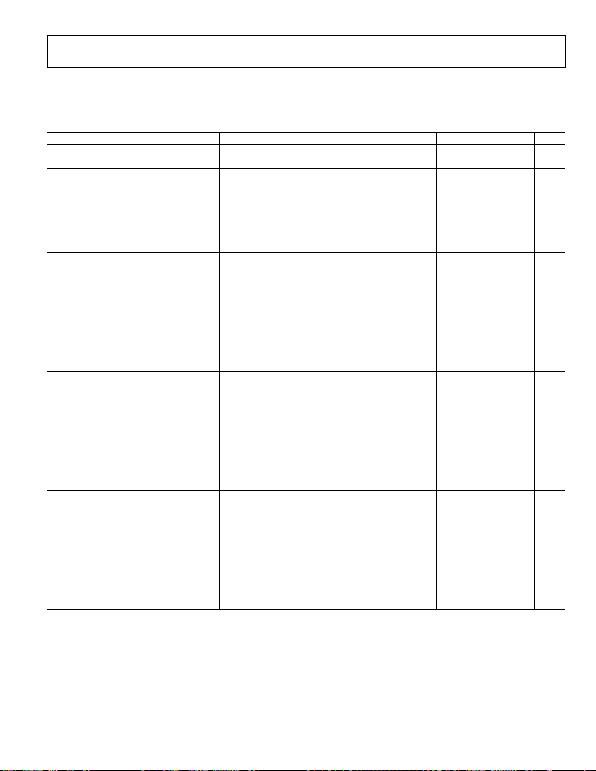Rev. A | Page 3 of 16
SPECIFICATIONS
VCC = 5 V and TA = 25°C, unless otherwise noted.
Table 1.
Parameter Conditions Min Typ Max Unit
OVERALL FUNCTION
Frequency Range
4000
MHz
FREQUENCY = 50 MHz
Gain
18.3
dB
Output 1 dB Compression Point (P1dB) 18.0 dBm
Output Third-Order Intercept (OIP3) Δf = 1 MHz, output power (POUT) = 0 dBm per tone 27.5 dBm
Second Harmonic POUT = 0 dBm −40.5 dBc
Third Harmonic POUT = 0 dBm −46.1 dBc
Noise Figure 2.9 dB
FREQUENCY = 140 MHz
Gain 17.0 dB
vs. Frequency ±50 MHz ±1.2 dB
vs. Temperature
−40°C ≤ T
A
≤ +85°C
±0.03
dB
vs. Supply Voltage 4.75 V to 5.25 V ±0.04 dB
Output 1 dB Compression Point (P1dB) 18.3 dBm
Output Third-Order Intercept (OIP3) Δf = 1 MHz, output power (POUT) = 0 dBm per tone 25.0 dBm
Second Harmonic POUT = 0 dBm −45.1 dBc
Third Harmonic
P
OUT
= 0 dBm
−55.1
dBc
Noise Figure 2.9 dB
FREQUENCY = 350 MHz
Gain
19.7
dB
vs. Frequency ±50 MHz ±0.20 dB
vs. Temperature −40°C ≤ TA ≤ +85°C ±0.31 dB
vs. Supply Voltage 4.75 V to 5.25 V ±0.01 dB
Output 1 dB Compression Point (P1dB) 20.0 dBm
Output Third-Order Intercept (OIP3) Δf = 1 MHz, output power (POUT) = 0 dBm per tone 36.5 dBm
Second Harmonic
P
OUT
= 0 dBm
−49.9
dBc
Third Harmonic POUT = 0 dBm −83.7 dBc
Noise Figure 3.0 dB
FREQUENCY = 700 MHz
Gain 19.0 20.2 21.0 dB
vs. Frequency ±50 MHz ±0.01 dB
vs. Temperature −40°C ≤ TA ≤ +85°C ±0.28 dB
vs. Supply Voltage 4.75 V to 5.25 V ±0.01 dB
Output 1 dB Compression Point (P1dB)
20.1
dBm
Output Third-Order Intercept (OIP3) Δf = 1 MHz, output power (POUT) = 0 dBm per tone 38.5 dBm
Second Harmonic POUT = 0 dBm −50.3 dBc
Third Harmonic POUT = 0 dBm −78.4 dBc
Noise Figure 3.0 dB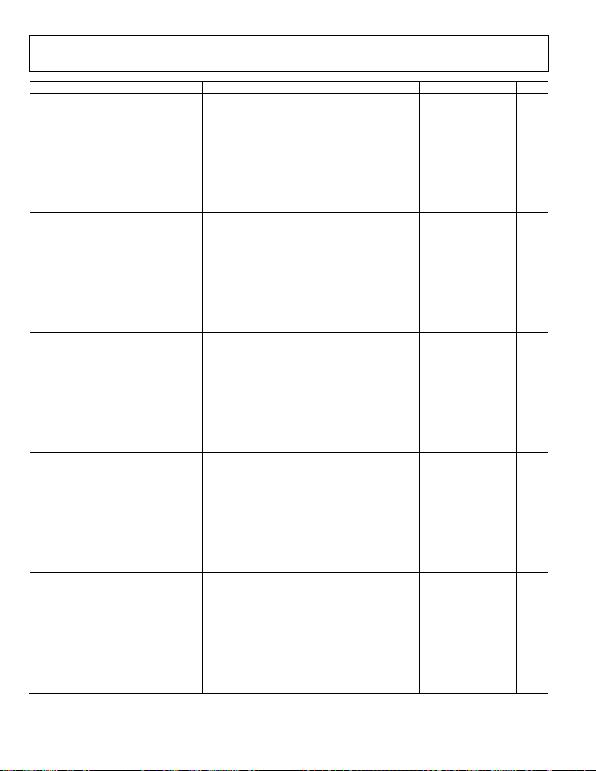Rev. A | Page 4 of 16
Parameter Conditions Min Typ Max Unit
FREQUENCY = 900 MHz
Gain 19.0 20.2 21.0 dB
vs. Frequency ±50 MHz ±0.01 dB
vs. Temperature
−40°C ≤ T
A
≤ +85°C
±0.28
dB
vs. Supply Voltage 4.75 V to 5.25 V ±0.01 dB
Output 1 dB Compression Point (P1dB) 19.0 20.1 dBm
Output Third-Order Intercept (OIP3) Δf = 1 MHz, output power (POUT) = 0 dBm per tone 40.0 dBm
Second Harmonic POUT = 0 dBm −59.4 dBc
Third Harmonic POUT = 0 dBm −77.3 dBc
Noise Figure 2.9 dB
FREQUENCY = 2000 MHz
Gain
19.5
dB
vs. Frequency ±50 MHz ±0.04 dB
vs. Temperature −40°C ≤ TA ≤ +85°C ±0.35 dB
vs. Supply Voltage 4.75 V to 5.25 V ±0.04 dB
Output 1 dB Compression Point (P1dB) 19.3 dBm
Output Third-Order Intercept (OIP3) Δf = 1 MHz, output power (POUT) = 0 dBm per tone 42.0 dBm
Second Harmonic POUT = 0 dBm −53.1 dBc
Third Harmonic POUT = 0 dBm −60.7 dBc
Noise Figure 3.3 dB
FREQUENCY = 2600 MHz
Gain 19.2 dB
vs. Frequency ±50 MHz ±0.01 dB
vs. Temperature
−40°C ≤ T
A
≤ +85°C
±0.28
dB
vs. Supply Voltage 4.75 V to 5.25 V ±0.05 dB
Output 1 dB Compression Point (P1dB) 18.7 dBm
Output Third-Order Intercept (OIP3) Δf = 1 MHz, output power (POUT) = 0 dBm per tone 36.5 dBm
Second Harmonic POUT = 0 dBm −52.8 dBc
Third Harmonic POUT = 0 dBm −67.4 dBc
Noise Figure 3.4 dB
FREQUENCY = 3500 MHz
Gain 19.3 dB
vs. Frequency
±50 MHz
±0.03
dB
vs. Temperature −40°C ≤ TA ≤ +85°C ±0.37 dB
vs. Supply Voltage 4.75 V to 5.25 V ±0.07 dB
Output 1 dB Compression Point (P1dB) 17.4 dBm
Output Third-Order Intercept (OIP3) Δf = 1 MHz, output power (POUT) = 0 dBm per tone 31.5 dBm
Second Harmonic POUT = 0 dBm −42.9 dBc
Third Harmonic POUT = 0 dBm −66.4 dBc
Noise Figure 3.8 dB
FREQUENCY = 4000 MHz
Gain 18.5 dB
vs. Frequency ±50 MHz ±0.19 dB
vs. Temperature −40°C ≤ TA ≤ +85°C ±0.73 dB
vs. Supply Voltage 4.75 V to 5.25 V ±0.08 dB
Output 1 dB Compression Point (P1dB) 15.2 dBm
Output Third-Order Intercept (OIP3) Δf = 1 MHz, output power (POUT) = 0 dBm per tone 28.0 dBm
Second Harmonic POUT = 0 dBm −44.1 dBc
Third Harmonic
P
OUT
= 0 dBm
−64.0
dBc
Noise Figure 4.2 dB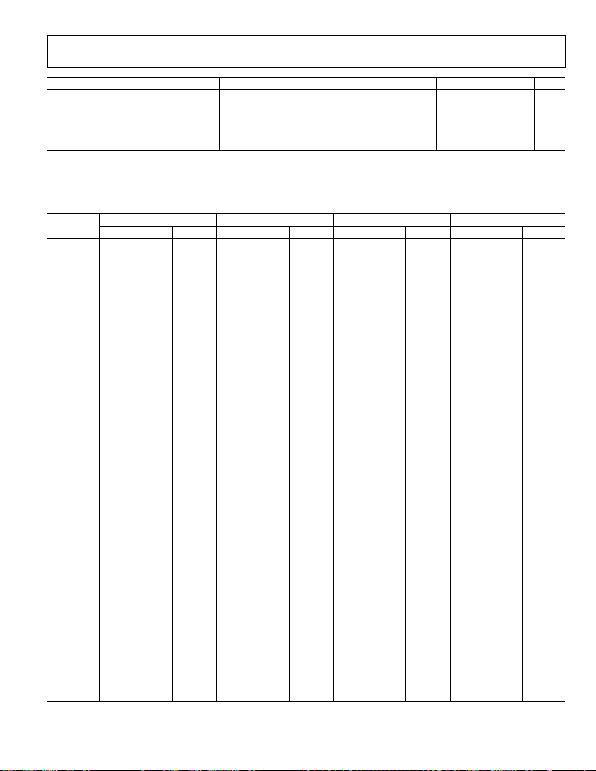Rev. A | Page 5 of 16
Parameter Conditions Min Typ Max Unit
POWER INTERFACE VCC
Supply Voltage (VCC) 4.5 5 5.5 V
Supply Current
89
106
mA
vs. Temperature −40°C ≤ TA ≤ +85°C ±3 mA
Power Dissipation VCC = 5 V 0.45 W
TYPICAL SCATTERING PARAMETERS (S PARAMETERS)
VCC = 5 V and TA = 25°C, the effects of the test fixture have been de-embedded up to the pins of the device.
Table 2.
Frequency
(MHz)
S11 S21 S12 S22
Magnitude (dB) Angle (°) Magnitude (dB) Angle (°) Magnitude (dB) Angle (°) Magnitude (dB) Angle (°)
50 −12.75 +32.81 18.14 +163.00 −25.27 −9.54 −8.96 −145.86
100 −8.98 −7.00 15.77 −179.92 −27.59 +4.56 −7.07 −178.96
150
−10.11
−34.04
17.27
−169.01
−26.11
+16.05
−7.89
+159.89
200 −11.97 −50.46 18.50 −168.73 −24.84 +16.55 −9.33 +147.22
250 −13.85 −62.42 19.21 −171.01 −24.14 +14.79 −10.87 +140.02
300 −15.69 −72.90 19.64 −173.77 −23.70 +12.89 −12.23 +136.16
350 −17.40 −81.30 19.90 −176.45 −23.43 +11.02 −13.56 +134.03
400 −19.17 −88.48 20.07 −178.86 −23.26 +9.65 −14.79 +132.79
450 −20.86 −97.45 20.18 +178.79 −23.14 +8.07 −15.99 +132.72
500 −22.52 −107.32 20.25 +176.86 −23.08 +7.11 −17.02 +133.33
550 −24.32 −112.89 20.32 +174.84 −23.00 +6.15 −18.21 +133.04
600 −26.07 −120.03 20.35 +173.09 −22.97 +5.24 −19.45 +135.06
650 −27.89 −128.79 20.39 +171.35 −22.93 +4.35 −20.73 +136.29
700
−30.79
−133.19
20.42
+169.67
−22.91
+3.72
−22.22
+136.96
750 −34.53 −136.03 20.42 +167.93 −22.91 +2.93 −23.91 +135.39
800 −42.59 −130.76 20.46 +166.48 −22.88 +2.30 −26.35 +136.07
850 −46.49 −2.93 20.47 +164.85 −22.88 +1.67 −29.40 +132.08
900 −34.26 +15.99 20.47 +163.17 −22.86 +0.98 −34.27 +116.48
950 −29.55 +9.83 20.48 +161.77 −22.86 +0.39 −40.86 +41.72
1000 −25.87 +5.52 20.48 +160.25 −22.86 −0.21 −31.44 −10.36
1050 −23.03 +1.85 20.47 +158.65 −22.89 −0.84 −26.19 −18.99
1100 −21.25 +0.10 20.45 +157.18 −22.90 −1.43 −23.27 −22.34
1150 −19.31 −1.89 20.45 +155.50 −22.92 −2.06 −20.70 −22.26
1200 −17.72 −4.09 20.40 +153.93 −22.96 −2.72 −18.67 −24.44
1250
−16.13
−6.53
20.35
+152.44
−23.04
−3.38
−16.92
−24.75
1300 −14.81 −8.71 20.31 +150.88 −23.07 −4.04 −15.44 −25.79
1350 −13.65 −10.01 20.24 +149.35 −23.16 −4.60 −14.14 −25.36
1400 −12.68 −11.33 20.18 +147.91 −23.22 −5.06 −13.12 −25.73
1450 −11.72 −12.65 20.11 +146.40 −23.32 −5.76 −12.04 −25.59
1500 −10.92 −13.56 20.04 +145.13 −23.39 −6.16 −11.20 −25.40
1550
−10.21
−14.37
19.97
+143.86
−23.49
−6.54
−10.49
−25.60
1600 −9.58 −15.37 19.88 +142.58 −23.57 −6.93 −9.77 −25.59
1650 −9.05 −15.74 19.80 +141.40 −23.69 −7.16 −9.21 −25.31
1700 −8.52 −16.73 19.72 +140.23 −23.76 −7.45 −8.66 −25.42
1750 −8.13 −17.13 19.64 +139.13 −23.87 −7.60 −8.26 −25.32
1800 −7.76 −17.59 19.57 +138.12 −23.94 −7.80 −7.91 −24.96
1850 −7.46 −17.76 19.50 +137.16 −24.05 −7.88 −7.56 −24.77
1900 −7.19 −17.92 19.43 +136.25 −24.13 −7.91 −7.35 −24.46
1950 −6.98 −17.84 19.39 +135.31 −24.18 −7.94 −7.13 −23.92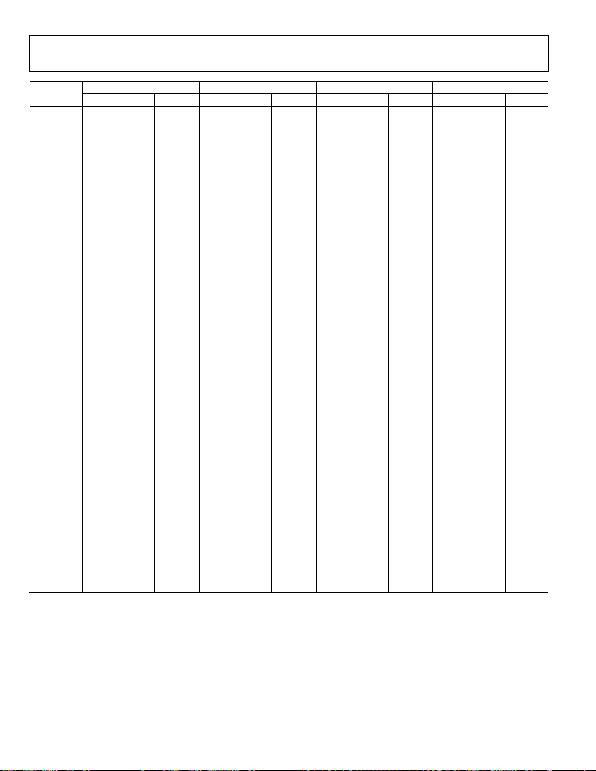Rev. A | Page 6 of 16
Frequency
(MHz)
S11 S21 S12 S22
Magnitude (dB) Angle (°) Magnitude (dB) Angle (°) Magnitude (dB) Angle (°) Magnitude (dB) Angle (°)
2000 −6.85 −18.00 19.35 +134.44 −24.26 −7.99 −6.96 −23.55
2050 −6.74 −17.93 19.31 +133.61 −24.29 −7.91 −6.86 −22.70
2100 −6.63 −17.57 19.29 +132.74 −24.37 −7.81 −6.75 −22.45
2150 −6.66 −17.34 19.25 +132.06 −24.42 −7.58 −6.76 −22.01
2200
−6.65
−17.26
19.29
+131.19
−24.40
−7.63
−6.78
−21.42
2250 −6.69 −17.10 19.30 +130.47 −24.41 −7.59 −6.82 −20.82
2300 −6.77 −16.90 19.33 +129.54 −24.42 −7.40 −6.87 −20.25
2350 −6.87 −16.65 19.36 +128.72 −24.40 −7.46 −7.04 −19.57
2400 −7.05 −16.25 19.42 +127.81 −24.38 −7.50 −7.20 −18.85
2450 −7.22 −16.11 19.49 +126.89 −24.35 −7.46 −7.42 −18.17
2500 −7.45 −16.00 19.53 +125.82 −24.31 −7.75 −7.66 −17.76
2550 −7.64 −15.84 19.58 +124.74 −24.31 −7.86 −7.91 −17.18
2600 −7.95 −15.66 19.66 +123.61 −24.25 −8.20 −8.29 −16.64
2650 −8.29 −15.64 19.74 +122.46 −24.19 −8.54 −8.66 −16.15
2700 −8.62 −15.95 19.81 +121.20 −24.17 −8.90 −8.97 −15.97
2750
−8.95
−15.92
19.89
+119.91
−24.13
−9.35
−9.41
−15.67
2800 −9.31 −16.23 19.94 +118.47 −24.08 −9.95 −9.87 −15.96
2850 −9.67 −16.74 20.03 +117.02 −24.05 −10.55 −10.34 −16.17
2900 −9.99 −17.33 20.07 +115.47 −24.07 −11.17 −10.83 −16.68
2950 −10.28 −18.00 20.14 +113.95 −24.02 −11.71 −11.28 −17.66
3000 −10.53 −19.16 20.18 +112.28 −24.03 −12.51 −11.69 −19.15
3050 −10.69 −20.29 20.21 +110.53 −24.10 −13.72 −12.17 −21.42
3100 −10.86 −21.94 20.25 +108.77 −24.04 −14.66 −12.54 −24.24
3150 −10.91 −23.61 20.27 +107.15 −24.09 −15.23 −12.83 −27.07
3200 −10.70 −25.42 20.28 +105.16 −24.18 −16.23 −12.92 −32.00
3250 −10.57 −27.33 20.28 +103.09 −24.17 −17.39 −13.04 −36.71
3300
−10.38
−29.60
20.26
+101.20
−24.22
−18.24
−12.99
−42.66
3350 −9.92 −31.76 20.21 +99.05 −24.39 −20.06 −12.57 −48.16
3400 −9.48 −34.25 20.15 +96.98 −24.49 −20.74 −12.19 −54.36
3450 −8.98 −36.47 20.08 +94.93 −24.61 −21.69 −11.64 −59.44
3500 −8.44 −38.18 20.00 +92.80 −24.72 −23.44 −11.00 −64.07
3550 −7.92 −40.32 19.90 +90.88 −24.88 −24.35 −10.34 −68.43
3600 −7.34 −42.20 19.74 +88.69 −25.15 −25.31 −9.59 −72.77
3650 −6.79 −43.72 19.57 +86.66 −25.35 −26.95 −8.90 −76.09
3700 −6.29 −45.15 19.41 +84.80 −25.56 −27.76 −8.29 −79.17
3750 −5.82 −46.51 19.23 +82.83 −25.90 −28.90 −7.69 −81.76
3800 −5.37 −47.51 19.00 +81.05 −26.16 −29.05 −7.12 −84.30
3850
−4.93
−48.36
18.78
+79.53
−26.46
−30.35
−6.65
−86.42
3900 −4.56 −48.94 18.55 +78.02 −26.81 −29.93 −6.20 −88.14
3950 −4.23 −49.33 18.32 +76.71 −27.08 −31.04 −5.83 −89.69
4000 −3.97 −49.71 18.08 +75.39 −27.50 −30.65 −5.50 −91.14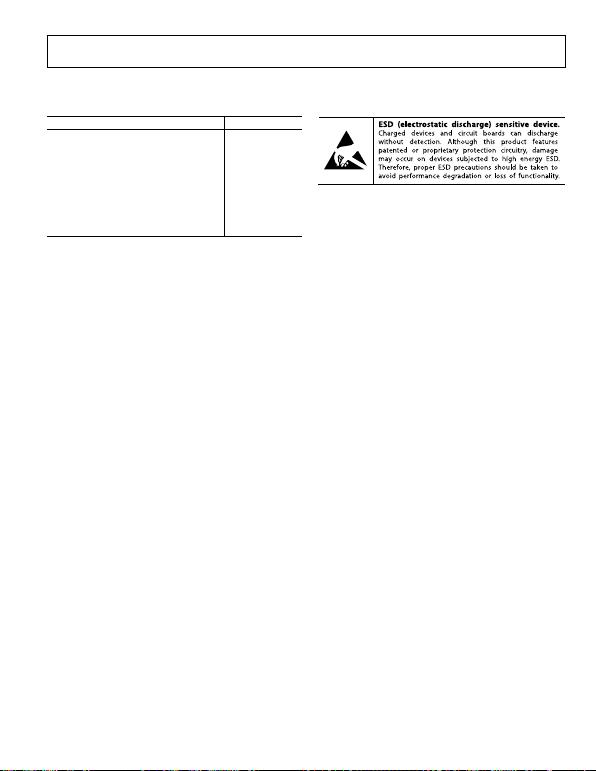Rev. A | Page 7 of 16
ABSOLUTE MAXIMUM RATINGS
Table 3.
Parameter Rating
Supply Voltage, VCC 6.5 V
Input Power (re: 50 Ω)
16 dBm
Internal Power Dissipation (Paddle Soldered) 600 mW
θJA (Junction to Air)
30.7°C/W
5.0°C/W
Maximum Junction Temperature 150°C
Lead Temperature (Soldering, 60 sec) 240°C
Operating Temperature Range
−40°C to +85°C
Storage Temperature Range −65°C to +150°C
Stresses above those listed under Absolute Maximum Ratings
may cause permanent damage to the device. This is a stress
rating only; functional operation of the device at these or any
other conditions above those indicated in the operational
section of this specification is not implied. Exposure to absolute
maximum rating conditions for extended periods may affect
device reliability.
ESD CAUTION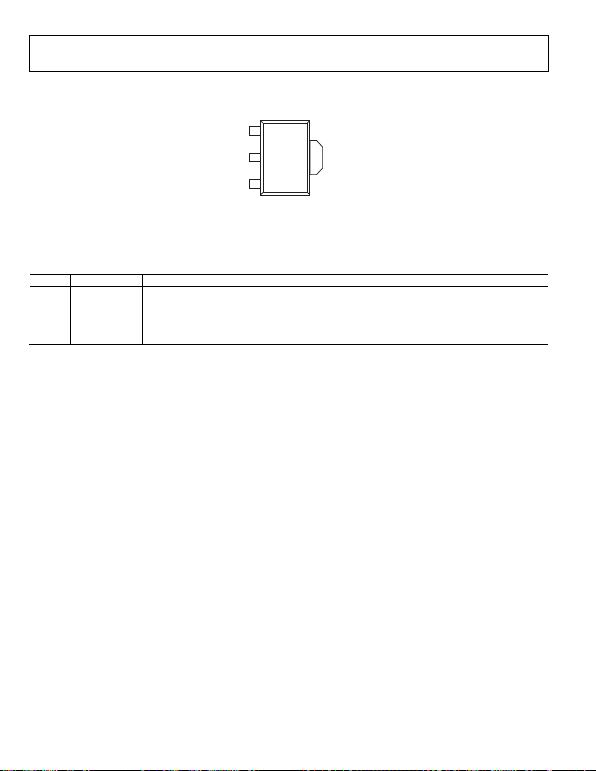Rev. A | Page 8 of 16
PIN CONFIGURATION AND FUNCTION DESCRIPTIONS
RFIN
GND
RFOUT
1
2
3
GND
TOP VI EW
(No t t o Scal e)
(2)
08190-002
NOTES
1. E X P OSED P ADDLE. I NTERNALLY
CONNECTED TO GND. SOLDER TO A
LOW IMP E DANCE GRO UND P LANE.
Figure 2. Pin Configuration
Table 4. Pin Function Descriptions
Pin No. Mnemonic Description
1
RFIN
RF Input. This pin requires a dc blocking capacitor.
2 GND Ground. Connect this pin to a low impedance ground plane.
3 RFOUT RF Output and Supply Voltage. DC bias is provided to this pin through an inductor that is connected to
the external power supply. The RF path requires a dc blocking capacitor.
(2) Exposed Paddle Exposed Paddle. Internally connected to GND. Solder to a low impedance ground plane.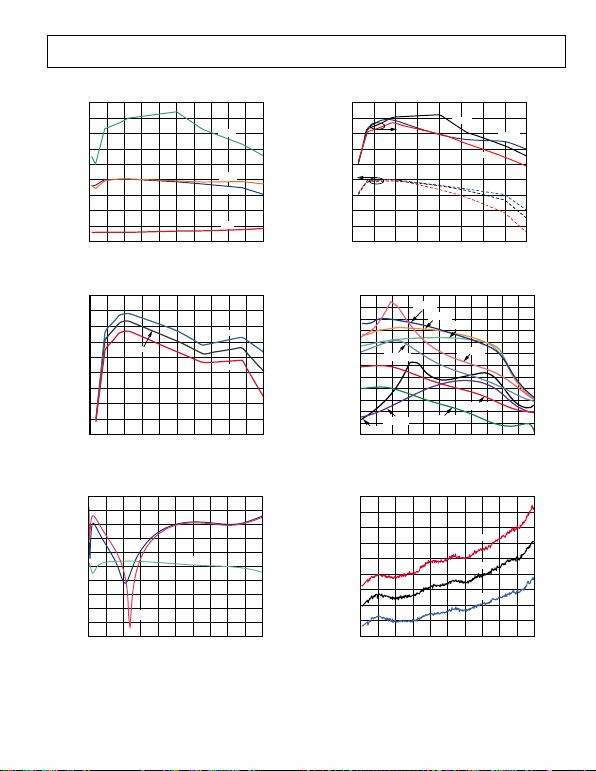Rev. A | Page 9 of 16
TYPICAL PERFORMANCE CHARACTERISTICS
0
5
10
15
20
25
30
35
40
45
0 0.4 0.8 1.2 1.6 2.0 2.4 2.8 3.2 3.6 4.0
NF, GAIN, P1dB, OIP3 (dB, dBm)
FREQUE NCY ( GHz)
OIP3
P1dB
GAIN
NF
08190-003
Figure 3. Noise Figure, Gain, P1dB, and OIP3 vs. Frequency
16.5
17.0
17.5
18.0
18.5
19.0
19.5
20.0
20.5
21.0
0 0.4 0.8 1.2 1.6 2.0 2.4 2.8 3.2 3.6 4.0
GAIN (dB)
FRE QUENC Y (GHz)
–40°C
+85°C
+25°C
08190-004
Figure 4. Gain vs. Frequency and Temperature
–50
–45
–40
–35
–30
–25
–20
–15
–10
–5
0
0 0.4 0.8 1.2 1.6 2.0 2.4 2.8 3.2 3.6 4.0
S-PARAMETERS ( dB)
FREQUENCY (G Hz )
S22
S12
S11
08190-005
Figure 5. Input Return Loss (S11), Output Return Loss (S22), and
Reverse Isolation (S12) vs. Frequency
0
5
10
15
20
25
30
35
40
45
12
14
16
18
20
22
24
26
28
30
0 0.5 1.0 1.5 2.0 2.5 3.0 3.5 4.0
OIP3 (dBm)
P1dB (dBm)
FREQUE NCY ( GHz)
–40°C
+85°C
+25°C
–40°C
+85°C
+25°C
08190-006
Figure 6. P1dB and OIP3 vs. Frequency and Temperature
20
22
24
26
28
30
32
34
36
38
40
42
44
5311357911131517
OIP3 (dBm)
P
OUT
PER T ONE (dBm)
0
8190-007
140MHz
50MHz
4000MHz
3500MHz
2600MHz 2000MHz
350MHz
700MHz
900MHz
Figure 7. OIP3 vs. Output Power (POUT) and Frequency
2.0
2.4
2.8
3.2
3.6
4.0
4.4
4.8
5.2
5.6
0 0.4 0.8 1.2 1.6 2.0 2.4 2.8 3.2 3.6 4.0
NOISE FI GURE (dB)
FRE QUENC Y (GHz )
+25°C
+85°C
–40°C
08190-008
Figure 8. Noise Figure vs. Frequency and Temperature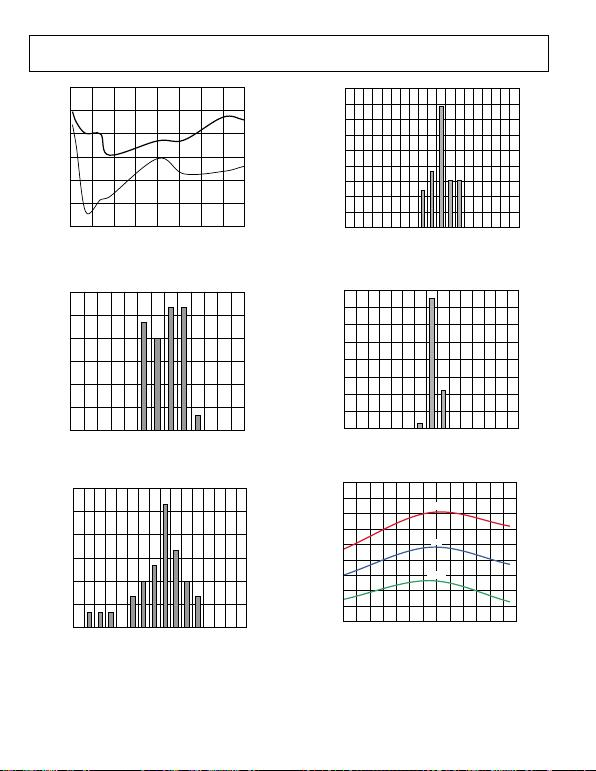Rev. A | Page 10 of 16
H2
H3
HARMO NICS (dBc)
–90
–80
–70
–60
–50
–40
–30
FREQUENCY (GHz)
0.5
01.0 1.5 2.0 2.5 3.0 3.5 4.0
08190-009
Figure 9. Single Tone Harmonics vs. Frequency , POUT = 0 dBm
0
5
10
15
20
25
30
18.8 19.0 19.2 19.4 19.6 19.8 20.0
PERCENTAG E ( %)
GAIN (d B)
08190-010
Figure 10. Gain Distribution at 2000 MHz
0
5
10
15
20
25
30
18.5 18.7 18.9 19.1 19.3 19.5 19.7 19.9
PERCENTAG E ( %)
P1d B ( dBm)
08190-011
Figure 11. P1dB Distribution at 2000 MHz
0
5
10
15
20
25
30
35
40
45
32 34 36 38 40 42 44 46 48 50
PERCENTAG E ( %)
OIP3 (dBm)
08190-020
Figure 12. OIP3 Distribution at 2000 MHz, POUT = 0 dBm
0
10
20
30
40
50
60
70
80
2.6 2.8 3.0 3.2 3.4 3.6 3.8 4.0
PERCE NTAGE ( %)
NOISE FIGURE (dB)
08190-021
Figure 13. Noise Figure Distribution at 2000 MHz
80
82
84
86
88
90
92
94
96
98
–40 –30 –20 –10 010 20 30 40 50 60 70 80 90
SUPPLY CURRENT ( mA)
TEMPERAT URE ( °C)
5.25V
4.75V
5V
08190-014
Figure 14. Supply Current vs. Temperature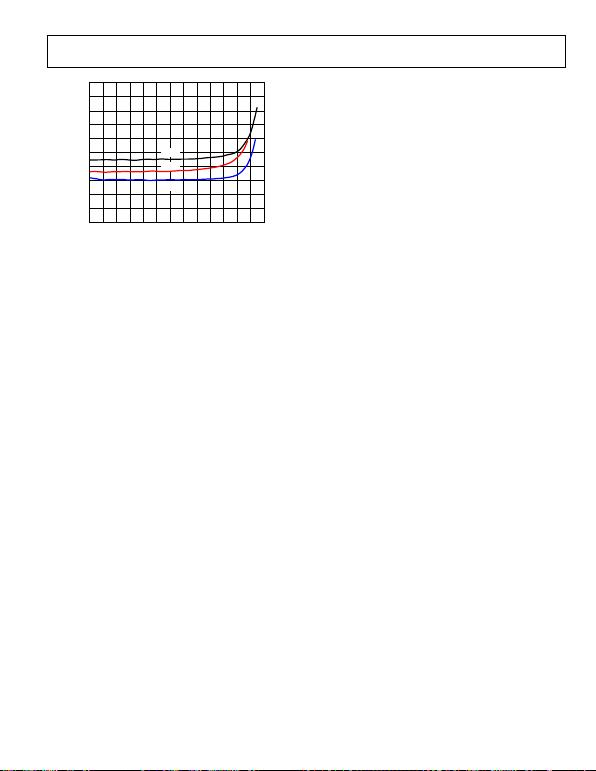Rev. A | Page 11 of 16
100
98
96
94
92
90
88
86
84
82
80–5 –3 –1 1357911 13 15 17 19 21
08190-100
SUPP LY CURRENT (mA)
P
OUT
(d Bm)
+25°C
–40°C
+85°C
Figure 15. Supply Current vs. POUT and Temperature VCC = 5 V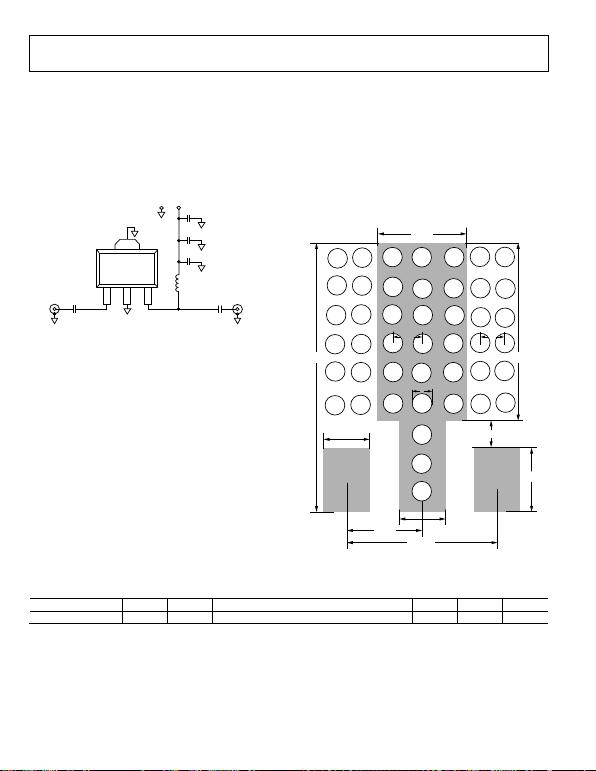Rev. A | Page 12 of 16
BASIC CONNECTIONS
The basic connections for operating the ADL5602 are shown in
Figure 16. Recommended components are listed in Table 5. The
input and output should be ac-coupled with appropriately sized
capacitors (device characterization was performed with 0.1 μF
capacitors). A 5 V dc bias is supplied to the amplifier through
the bias inductor connected to RFOUT (Pin 3). The bias voltage
should be decoupled using a 1 µF capacitor, a 1.2 nF capacitor,
and a 68 pF capacitor.
RFIN
GND
GND
RFOUT
123
C6
1µF
L1
470nH
GND
RFIN
RFOUT
(2)
C5
1.2nF
C4
68pF
C2
0.1µF
C1
0.1µF
VCC
08190-015
Figure 16. Basic Connections
SOLDERING INFORMATION AND RECOMMENDED
PCB LAND PATTERN
Figure 17 shows the recommended land pattern for the ADL5602.
To minimize thermal impedance, the exposed paddle on the
package underside should be soldered down to a ground plane
along with Pin 2. If multiple ground layers exist, they should be
pattern design and layout, refer to the AN-772 Application
Note, A Design and Manufacturing Guide for the Lead Frame
Chip Scale Package (LFCSP).
1.50mm
3.00mm
1.27mm
0.62mm
3.48mm
1.80mm
0.86mm
5.37mm
0.20mm
0.762mm 0.635mm
0.86mm
08190-101
Figure 17. Recommended Land Pattern
Table 5. Recommended Components for Basic Connections
Frequency (MHz) C1 C2 L1 C4 C5 C6
50 to 4000 0.1 µF 0.1 µF 470 nH (Coilcraft 0603LS-NX or equivalent) 68 pF 1.2 nF 1 µF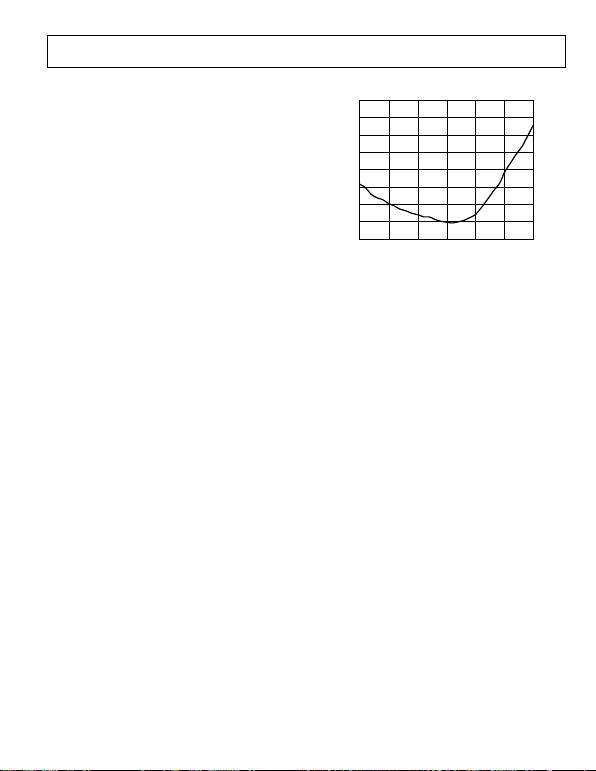Rev. A | Page 13 of 16
W-CDMA ACPR PERFORMANCE
Figure 18 shows a plot of adjacent channel power ratio (ACPR)
vs. POUT for the ADL5602. The signal type being used is a single
W-CDMA carrier (Test Model 1-64) at 2140 MHz. This signal is
generated by a very low ACPR source. ACPR is measured at the
output by a high dynamic range spectrum analyzer, which
incorporates an instrument noise correction function.
The ADL5602 achieves an ACPR of −75 dBc at −5 dBm output,
at which point device noise and not distortion is beginning to
dominate the power in the adjacent channels. At an output
power of +5 dBm, ACPR is still very low at −61 dBc, making
the device particularly suitable for PA driver applications.
–40
–45
–50
–55
–60
–65
–70
–75
–80
–20 –15 –10 –5 0 5 10
ACPR AT 5MHz CARRIER OF FSE T (d Bc)
P
OUT
(d Bm)
08190-017
Figure 18. ACPR vs. POUT, Single Carrier W-CDMA (Test Model 1-64) at
2140 MHz Evaluation Board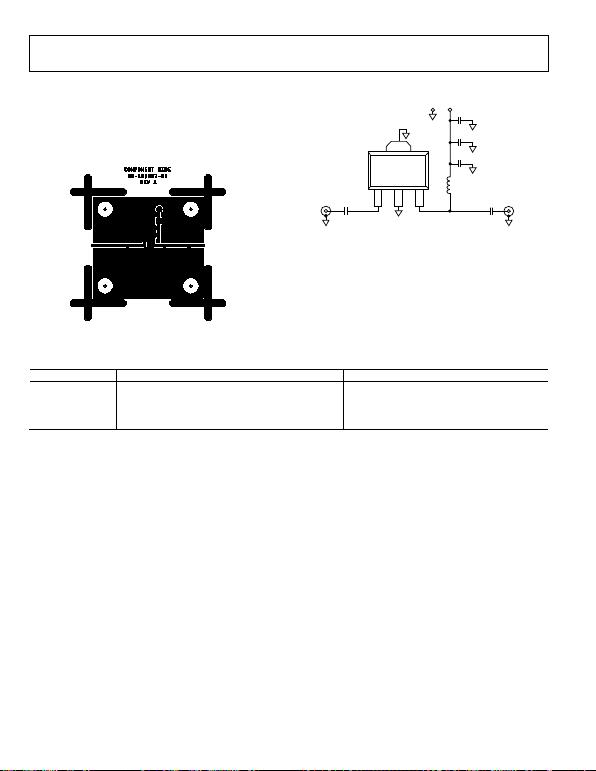Rev. A | Page 14 of 16
EVALUATION BOARD
Figure 20 shows the schematic for the ADL5602 evaluation
The components used on the board are listed in Table 6.
Power can be applied to the board through clip-on leads
(VCC and GND).
08190-018
Figure 19. Evaluation Board Layout (Top)
RFIN
GND
GND
RFOUT
123
C6
1µF
L1
470nH
GND
RFIN
RFOUT
(2)
C5
1.2nF
C4
68pF
C2
0.1µF
C1
0.1µF
VCC
08190-019
Figure 20. Evaluation Board Schematic
Table 6. Evaluation Board Configuration Options
Component Description Default Value
C1, C2 AC-coupling capacitors 0.1 μF, 0402
L1 DC bias inductor 470 nH, 0603 (Coilcraft 0603LS-NX or equivalent)
VCC and GND Clip-on terminals for power supply
C4, C5, C6 Power supply decoupling capacitors C4 = 68 pF, 0603; C5 = 1.2 nF, 0603; C6 = 1 μF, 1206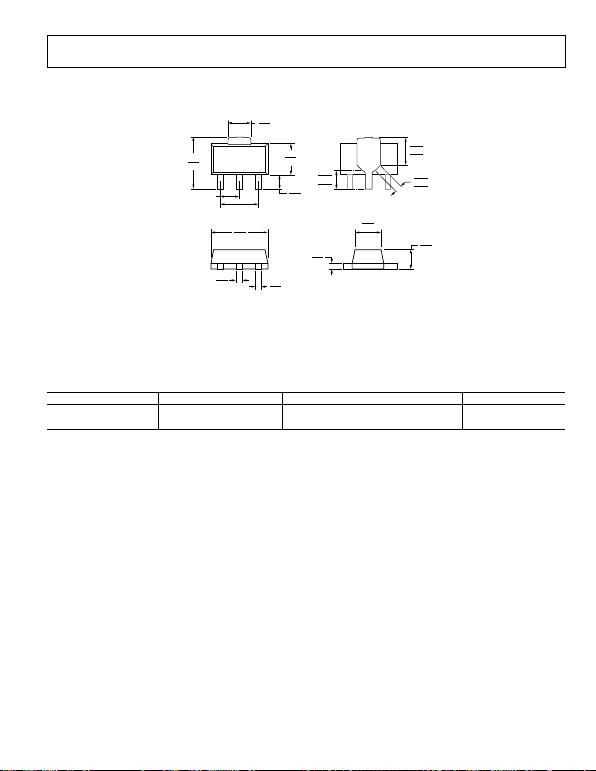Rev. A | Page 15 of 16
OUTLINE DIMENSIONS
COM P LIANT T O JEDE C S TANDARDS TO- 243
4.25
3.94
4.60
4.40
1.75
1.55
1.50 TYP
3.00 TYP
END VIEW
2.60
2.30
1.20
0.75
1 2
(2)
3
2.29
2.14
0.56
0.36 0.52
0.32
1.60
1.40
0.44
0.35
TOP VIEW
BOTTOM VIEW
2.413
2.380
2.337
1.270
1.252
1.219
0.635
0.569
0.508
09-12-2013-C
PKG-003480
Figure 21. 3-Lead Small Outline Transistor Package [SOT-89]
(RK-3)
Dimensions shown in millimeters
ORDERING GUIDE
Model1 Temperature Range Package Description Package Option